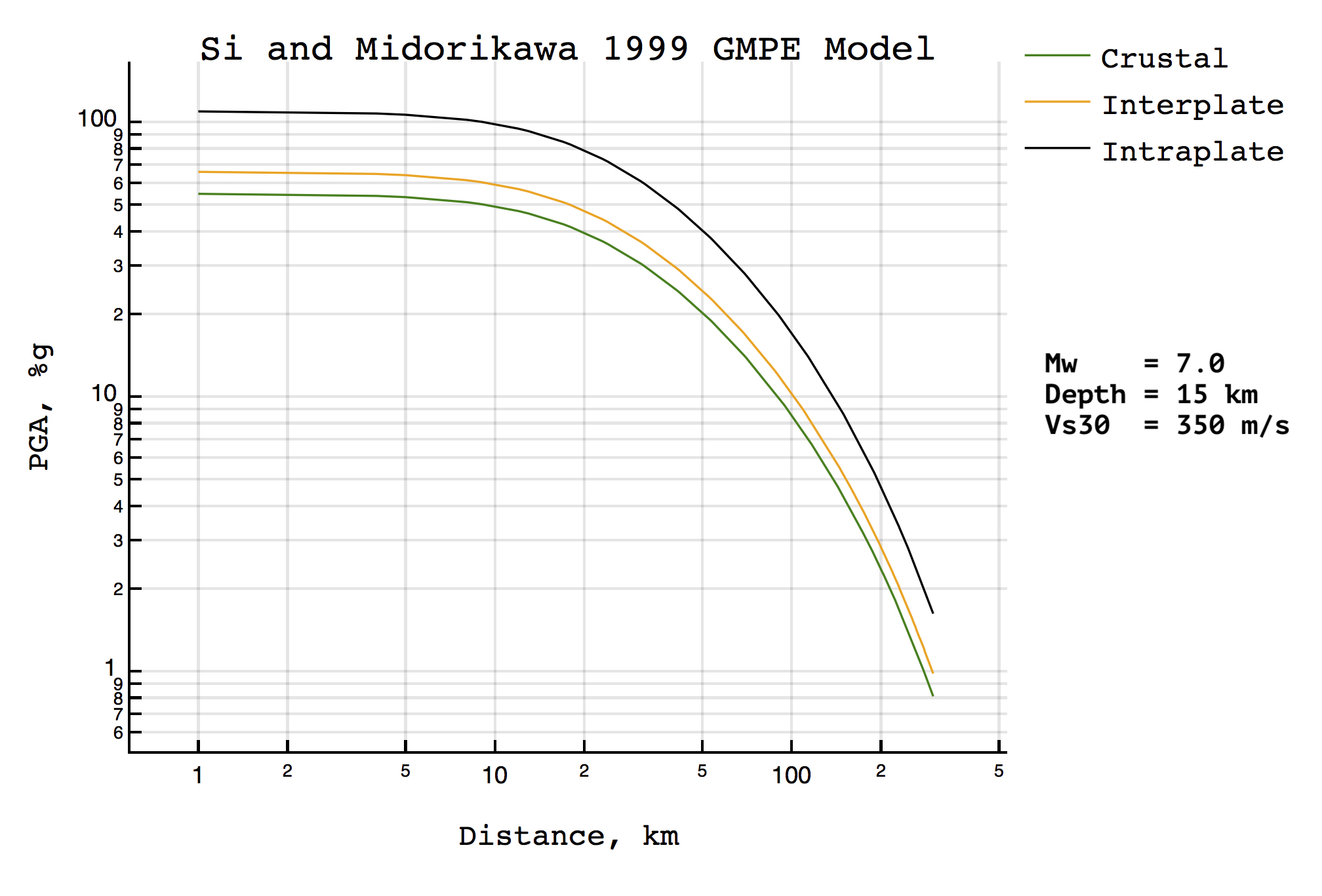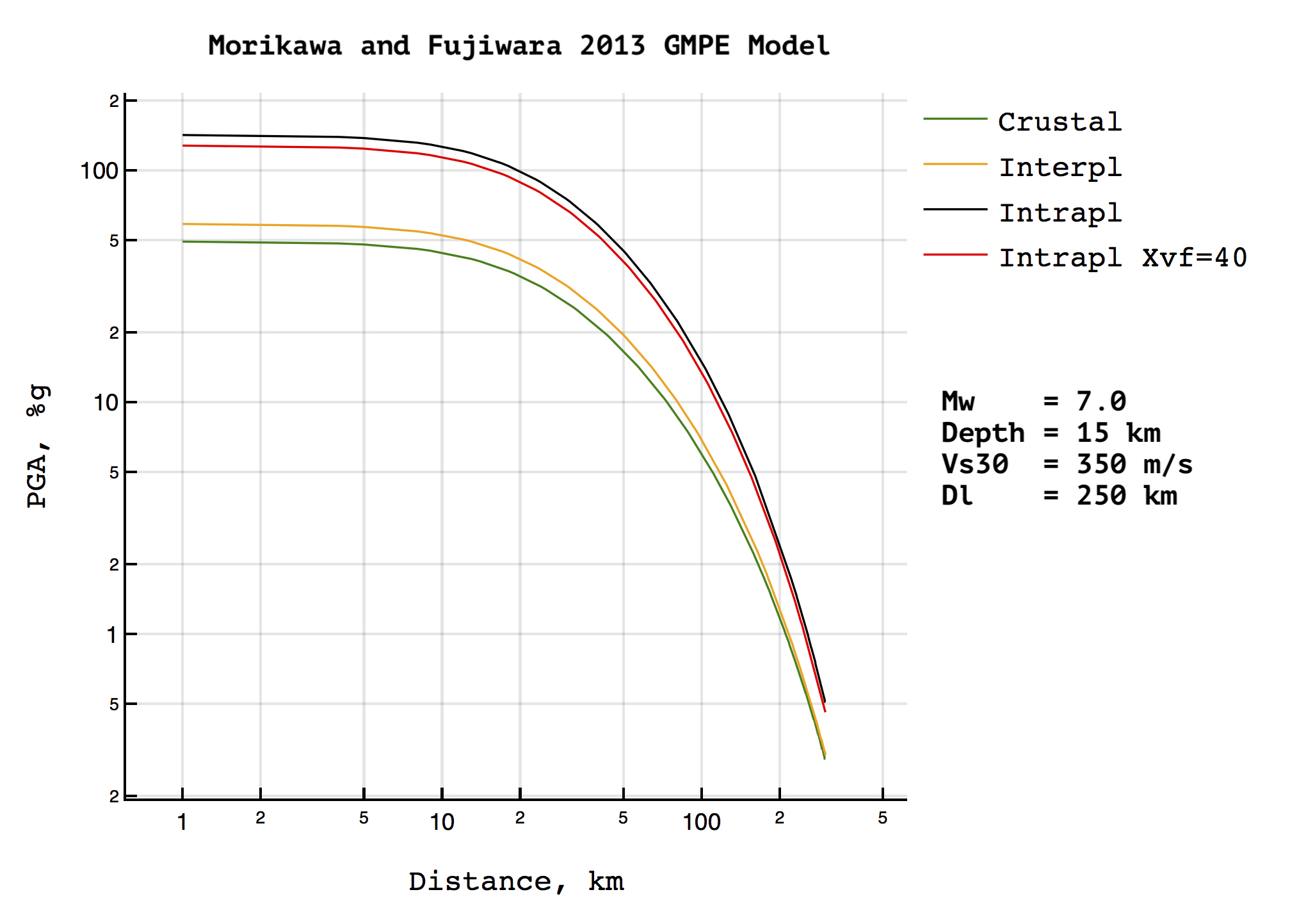1

0

1

1

# GroundMotion.jl

The ground motion evaluation module (earthquake seismology)

### Install

``````(v1.0) pkg> add GroundMotion.jl
``````

## Basic principles

Names of GMPE functions looks like: `gmpe_{Name_of_gmpe_function}`. For example: `gmpe_as2008`, where `as2008` is Abrahamson and Silva 2008 GMPE Model. The configuration for a model (see `examples/*.conf`) has `ground_motion_type` that can be `PGA`,`PGV`,`PSA` and define the type of output data points.

Each GMPE function has at least 2 methods: for calculation based on input VS30-grid or without any grid.

### GRID case

The GMPE function for each grid's point calculates `{pga/pgv/psa}` values using `latitude`, `longitude` [degrees for WGS84 ellipsoid] and `VS30` [m/s]. The output data has return in custom type (depends by config) where latitude and longitude are copy from input grid and `pga/pgv/pgd/psa` is calculated by function.

For example: the function `gmpe_as2008` with parameters

``````pga_as2008(eq::Earthquake,
config_as2008::Params_as2008,
grid::Array{Point_vs30};
min_val::Number)
``````

where `ground_motion_type = "PGA"` at `config`, returns 1-d is `Array{Point_pga_out}` with points based on input grid and `pga > min_val` (`pga` is Acceleration of gravity in percent (%g) rounded to `ggg.gg`).

### Without grid

In case of without any grid GMPE functions return simple 1-d `Array{Float64}` with `{pga/pgv/pgd/psa}` data. It calculates from epicenter to `distance` with `1` [km] step perpendicularly to the epicenter.

Example:

``````pga_as2008(eq::Earthquake,
config::Params_as2008;
VS30::Number=350,
distance::Int64=1000)
``````

where `ground_motion_type = "PGA"` at `config`, return is `Array{Float64}` with `1:distance` values of `pga` (also rounded to `ggg.gg`).

## Short example:

``````using GroundMotion
# init model parameters
include("GroundMoution.jl/examples/as2008.conf")
# set earthquake location
eq = Earthquake(143.04,51.92,13,6)
# run AS2008 PGA modeling on GRID
out_grid = gmpe_as2008(eq,config_as2008,grid)
# run AS2008 PGA FOR PLOTTING with VS30=30 [m/s^2], distance=1000 [km] by default.
simulation = pga_as2008(eq,config_as2008)
``````

## How to get VS30 grid

1. Download GMT grd file from USGS Vs30 Models and Data page
2. Unzip it. It takes around 2,7G disk space for one file: ``` unzip global_vs30_grd.zip ... ls -lh global_vs30.grd -rw-r--r-- 1 jamm staff 2,7G 8 сен 2016 global_vs30.grd ```
3. Use `GMT2XYZ` man page from GMT to convert grd data to XYZ text file: ``` # example: grd2xyz global_vs30.grd -R145.0/146.0/50.0/51.0 > test_sea.txt # number of rows: cat test_sea.txt |wc -l 14641 ```

## Read and Write data grids

Use `read_vs30_file` to read data from vs30 file:

``````grid = read_vs30_file("Downloads/web/somevs30.txt")
``````

After some `gmpe_*` function on grid done, you will get `Array{Point_{pga,pgv,pgd,psa}_out}`. Use `convert_to_float_array` to convert `Array{Point_{pga,pgv,pgd,psa}_out}` to `Nx3` `Array{Float64}`:

``````typeof(A)
#--> Array{GroundMoution.Point_pga_out,1}
length(A)
#--> 17
B = convert_to_float_array(A)
typeof(B)
#--> Array{Float64,2}
``````

Use `Base.writedlm` to write XYZ (`lon`,`lat`,`pga/pgv/pgd/psa`) data to text file:

``````writedlm("Downloads/xyz.txt", B) # where B is N×3 Array{Float64}
``````

Use `convert_to_point_vs30` to convert Array{Float64,2} array to Array{GroundMotion.Point_vs30,1}

## Earthquake location data

Lets define `lat`,`lon`,`depth`,`Ml`,`Mw`:

``````eq = Earthquake(143.04,51.92,13,6,5.8)
# OR
eq = Earthquake(143.04,51.92,13,6)
``````

Latitude and longitude assumes degrees for WGS84 ellipsoid. Depth in km. `Mw` usually not ready right after earthquake. `Mw=0` in case of moment magnitude is not specified. All gmpe models uses `Mw` if it is or `Ml` otherwise.

## Abrahamson and Silva 2008 GMPE Model

WORK IN PROGRESS!

### Reference

Abrahamson, Norman, and Walter Silva. "Summary of the Abrahamson & Silva NGA ground-motion relations." Earthquake spectra 24.1 (2008): 67-97.

### PGA:

``````## ON GRID
gmpe_as2008(eq::Earthquake,
config_as2008::Params_as2008,
grid::Array{Point_vs30};
min_val::Number)
## Without grid
gmpe_as2008(eq::Earthquake,
config::Params_as2008;
VS30::Number=350,
distance::Int64=1000)
``````

Keyword arguments: `min_val`,`VS30`,`distance`.

### Model Parameters

See `examples/as2008.conf`.

The variables that always zero for current version:

`a12*Frv`, `a13*Fnm`, `a15*Fas`, `Fhw*f4(Rjb,Rrup,Rx,W,S,Ztor,Mw)`, `f6(Ztor)`, `f10(Z1.0, Vs30)`.

Actually they are not presented at code.

R_rup - is a distance to hypocenter

## Si and Midorikawa 1999 GMPE Model### References

1. Si, Hongjun, and Saburoh Midorikawa. "New attenuation relations for peak ground acceleration and velocity considering effects of fault type and site condition." Proceedings of twelfth world conference on earthquake engineering. 2000.
2. Si H., Midorikawa S. New Attenuation Relationships for Peak Ground Acceleration and Velocity Considering Effects of Fault Type and Site Condition // Journal of Structural and Construction Engineering, A.I.J. 1999. V. 523. P. 63-70, (in Japanese with English abstract).

### PGA:

``````## ON GRID
gmpe_simidorikawa1999(eq::Earthquake,
config::Params_simidorikawa1999,
grid::Array{Point_vs30};
min_val::Number)
## Without grid
gmpe_simidorikawa1999(eq::Earthquake,
config::Params_simidorikawa1999;
VS30::Number=350,
distance::Int64=1000)
``````

Keyword arguments: `min_val`,`VS30`,`distance`.

### Model Parameters

See `examples/si-midorikawa-1999.conf`.

X - is a distance to hypocenter

## Morikawa and Fujiwara 2013 GMPE Model### Reference

Morikawa N., Fujiwara H. A New Ground Motion Prediction Equation for Japan Applicable up to M9 Mega-Earthquake // Journal of Disaster Research. 2013. Vol. 5 (8). P. 878–888.

### PGA, PGV, PSA

``````## On grid whithout Dl data
gmpe_mf2013(eq::Earthquake,
config::Params_mf2013,
grid::Array{Point_vs30};
min_val::Number=0,
Dl::Number=250,
Xvf::Number=0)
## On grid with Dl data
gmpe_mf2013(eq::Earthquake,
config::Params_mf2013,g
rid::Array{Point_vs30_dl};
min_val::Number=0,
Xvf::Number=0)
## without any grid
gmpe_as2008(eq::Earthquake,
config::Params_mf2013;
VS30::Number=350,
distance::Int64=1000,
Dl::Number=250,
Xvf::Number=0)
``````

`min_val=0`, `Xvf=0` [km] by default. `Dl=250` [km] by default in case of grid pass without Dl data.

NOTE that `gmpe_mf2013` has next keyword arguments: `min_val`, `min_val`, `Dl`, `VS30`, `distance`. The keyword arguments should be pass with name. Example: `gmpe_mf2013(eq,config,VS30=500,Xvf=40)`.

### Model Parameters

See `examples/morikawa-fujiwara-2013.conf`

The `Dl` is the top depth to the layer whose S-wave velocity is `l` (in `[m/s]`) at the site. Actually it should be another one grid with `Dl` depths on each grid point (`Point_vs30_dl` type). If you pass grid without `Dl`, then `Dl` variable pass to GMPE functions as a constant.

X - is a distance to hypocenter

Licensed under the Apache License, Version 2.0 (the "License"); you may not use this file except in compliance with the License. You may obtain a copy of the License at

``````   http://www.apache.org/licenses/LICENSE-2.0
``````

Unless required by applicable law or agreed to in writing, software distributed under the License is distributed on an "AS IS" BASIS, WITHOUT WARRANTIES OR CONDITIONS OF ANY KIND, either express or implied. See the License for the specific language governing permissions and limitations under the License.

06/20/2017

8 months ago

92 commits